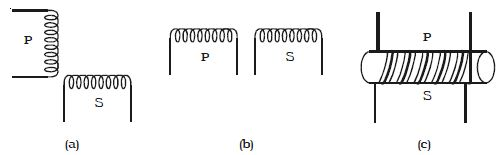Physics

# Explain Coefficient of Mutual Induction

The coefficient of mutual induction of two coils is numerically equal to the emf induced in one coil when the rate of change of current through the other coil is unity. The unit of the coefficient of mutual induction is Henry.

One Henry is defined as the coefficient of mutual induction between a pair of coils when a change of current of one ampere per second in one coil produces an induced emf of one volt in the other coil.

The coefficient of mutual induction between a pair of coils depends on the following factors

(i) Size and shape of the coils, number of turns and permeability of material on which the coils are wound.

(ii) the proximity of the coils

Two coils P and S have their axes perpendicular to each other (Fig: a). When a current is passed through coil P, the magnetic flux linked with S is small and hence, the coefficient of mutual induction between the two coils is small.

The two coils are placed in such a way that they have a common axis (Fig: b). When current is passed through the coil P the magnetic flux linked with coil S is large and hence, the coefficient of mutual induction between the two coils is large.If the two coils are wound on a soft iron core (Fig: c) the mutual induction is very large.